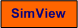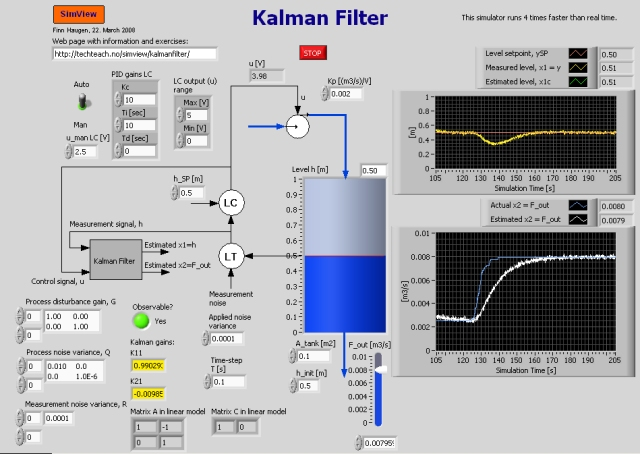# Kalman Filter

Snapshot of the front panel of the simulator:## Aims

The aim of this simulator is to show how a Kalman Filter can be used to continuously estimate the value of an unknown process variable. The typical trade-off is demonstrated: You can not have both fast estimate update and noise-attenuated estimates, i.e. you have to choose to emphasize either of them.

## Motivation

The Kalman Filter is a generic algorithm for estimating process variables, assuming that you have a mathematical model of the process (a set of differential equations) and that there is at least one variable that is continuously measured. The Kalman Filter is used in a large variety of applications - in control applications where the controller uses estimates as if they were real measurements and in supervisory systems (as "soft-sensors").

## Description of the simulated system

The process is a water tank with inflow and outflow. The level is maintained at or close to a level setpoint by a level controller which controls the inflow. The outflow, which is regarded as a disturbance to the process, can be adjusted freely by the user. A Kalman Filter is used to estimate the assumed unknown outflow. Detailes about the model and the Kalman Filter are described in kalmanfilter.pdf.